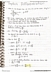# MATH 1151 Study Guide - Midterm Guide: Asymptote, Electrical Contacts, University Of Manchester

36 views10 pages
School
Department
Course
Professorelden1340 and 38522 others unlocked75
Verified Note
75 documents

## Document Summary

The graph of a function f is given in the figure below. Use the graph of f to answer the questions below. Hnote : possible answers include + , - , or "does not exist". l fhxl = 5. Form a, page 3 x -2 x - x + lim lim x -2+ x -2- fhxl = + fhxl = 0 fhxl = does not exist fhxl = 0 fhxl = + . Hvilh4 ptsl determine the intervals of continuity for f . H- , -2l , @-2, 3^ , and h3, + l. Find all slant asymptotes. x = - 2 y = 0 y = x + 2. Use a right triangle to simplify the given expression. T herefore, tani cos-1 xm = tan q = Find the inverse of the given function and write it in the form y =